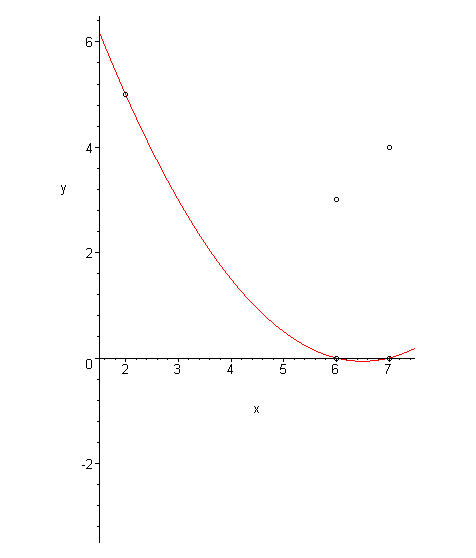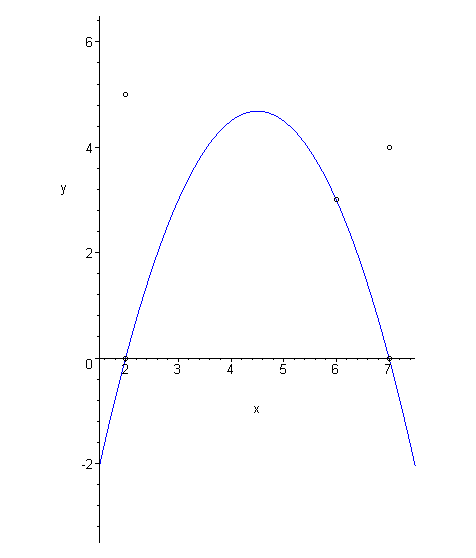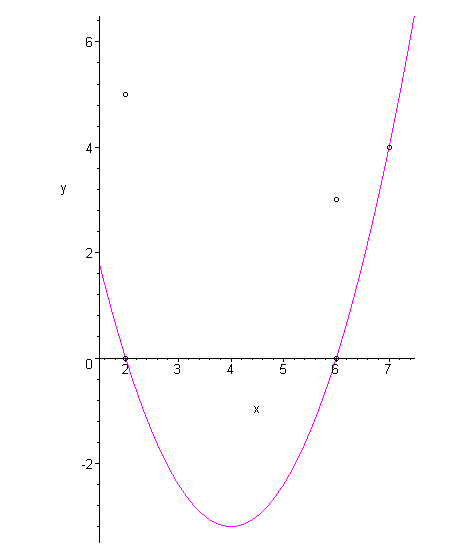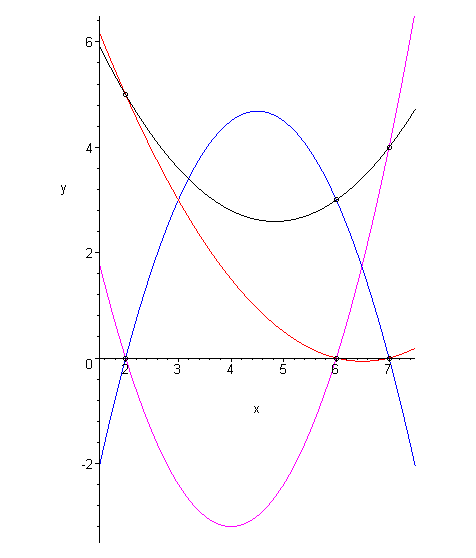# Introduction

The Vandermonde method is an appropriate computation method for finding interpolating polynomials. The method of Lagrange polynomials is more intuitive and and may be more easily calculated by hand.

# Background

Useful background for this topic includes:

• Polynomials

# Theory

The Vandermonde method gives a simple mechanical technique for finding interpolating polynomials, however, it is not very convenient for paper problems. The method of Lagrange polynomials gives an intuitive method of finding interpolating polynomials (usually for a very small number of points, say two to four).

The technique is relatively simple: to find the interpolating polynomial which passes through the points (x1, y1), ..., (xn, yn), find n polynomials according to these rules:

• The first polynomial equals y1 at x1 and equals zero at x2, ..., xn,
• The second polynomial equals y2 at x2 and equals zero at x1, x3, ..., xn,

• The nth polynomial equals yn at xn and equals zero at x1, ..., xn - 1,

Constructing these polynomials is quite easy. Consider the polynomial

This polynomial is zero at the points x2, ..., xn, but it equals 1 at x1 (the numerator cancels out the denominator at that point).

Similarly,

equals zero at all other points except for x2 where it equals 1.

We may define such a function for all n points up to:

which equals zero at all points other than xn where it equals 1.

If we calculate the define

then it follows that p(xi) = yi for i = 1, 2, ..., n. Thus, p is a polynomial which interpolates the given n points.

# HOWTO

While the theory section describes how to generate an arbitrary Lagrange polynomial, this section will focus on two cases: n = 2 and n = 3.

# Problem

Find an interpolating polynomial which passes through two or three points.

# Assumptions

The xi's are unique.

# Process: n = 2

To find the Lagrange polynomial which interpolates the three points (x1, y1) and (x2, y2), define:

and add: y1 L1(x) + y2 L2(x) to get

# Process: n = 3

To find the Lagrange polynomial which interpolates the three points (x1, y1), (x2, y2), and (x3, y3), define:

and add: y1 L1(x) + y2 L2(x) + y3 L3(x) to get

# Error Analysis

The error associated with Lagrange polynomials is the same as that for the Vandermonde method.

# Example 1

Find the Lagrange polynomial which interpolates the points (2, 4), (3, 1), (5, 7).

The polynomial is:

which simplifies to

# Example 2

Find the Lagrange polynomial which interpolates the points (1, 2), (3, 4).

Using Lagrange polynomials to find interpolating functions for two points is very easy: 2 (x − 3)/(1 − 3) + 4 (x − 1)/(3 − 1) = −(x − 3) + 2 (x − 1).

# Example 3

Sketch the three Lagrange polynomials which are added to find the interpolating polynomial of the three points (2, 5), (6, 3), (7, 4).

Figure 1 shows the first Lagrange polynomial which equals 5 at x = 2 and zero at 6 and 7, Figure 2 shows the second Lagrange polynomial which equals 3 at x = 6 and zero at 2 and 7, and Figure 3 shows the third Lagrange polynomial which equals 4 at x = 7 and zero at 2 and 6.Figure 1. The first Lagrange polynomial: zero at x = 6 and x = 7.Figure 2. The second Lagrange polynomial: zero at x = 2 and x = 7.Figure 3. The third Lagrange polynomial: zero at x = 2 and x = 6.

The sum of these polynomials is shown in Figure 4.Figure 4. The sum of the three Lagrange polynomials.

# Question 1

Find the Lagrange polynomial which interpolates the points (2, 9), (3, -14), (5, -24).

Answer: p(x) = 3(x − 3)(x − 5) + 7(x − 2)(x − 5) − 4(x − 2)(x − 3).

# Question 2

Find the Lagrange polynomial which interpolates the points (0, 1), (1, 0), (3, 4).

Answer: p(x) = 1/3 (x − 1)(x − 3) + 0 + 2/3 x(x − 1)

# Question 3

Show that the polynomial found in Question 2 is the same polynomial found using the Vandermonde method.

# Question 4

Find the Lagrange polynomial which interpolates the two points (5.3, 4.6) and (7.3, 2.6).

Answer: p(x) = -2.3 (x − 7.3) + 1.3 (x − 5.3)

# Question 5

Sketch the four Lagrange polynomials which are added to find the interpolating polynomial which passes through the four points (1, 4), (2, 7), (5, 6), (8, 3).

# Applications to Engineering

Lagrange polynomials are useful when trying to quickly determine interpolating polynomials by hand. They are appropriate for back-of-the-envelope calculations.

# Matlab

As the method of Lagrange polynomials is not suited towards numeric computation, it is not implemented in Matlab nor is it an easy task to do so.

# Maple

Finding an interpolating polynomial with Maple may be done with the PolynomialInterpolation routine:

```CurveFitting[PolynomialInterpolation]( [[0.3, 1.5], [0.4, 1.7], [0.6, 2.5]], x, form = lagange );
plot( %, x = 0..1 );
```

For more help on either of this routine or on the CurveFitting package, enter:

```?CurveFitting
?CurveFitting,PolynomialInterpolation
```